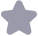收藏

midx=0.5米(×1+×2) midy=0.5米(y1+y2) if((-RESOLUtION<(bulge-1))&&((bulge-1)<RESOLUTION)) printf("arc:(x=%f y=%f r=%f )n", midx, midy, radius) parc->cy=midy parc->cz=0.⊙; parc->r=radiUS printf(x=%Lf, y=%Lf, z=%f r=%f\n", parc->Cx, parc->cy, parc->CZ, parc->r) turn 1 //printf( mid=%f mid=%f \ n", midx, midy) //弦矢量的方向角(-2P之) double chordangle=o;//起点到终点的弦向量与x正方向之间的倾斜角 chordangleacos((x2-x1)sgrt( pow(x2-X1, 2)+pow(y2-y1, 2))) printf ("chordangLe =%f n", chordangle) double amass;/弦向量与X轴正向单位向量的叉积=(y2-y1)k anas5=y2-91;//由(由(x2-×1)*-1米(g2-y1))得到 if(amass<o) chordang le=2*PI-chordangLe: // printf("here、n") printf(" chordangLe =%f \n", chordangLe*180/PI) double DirectionAngle=0,.0:/弦中点到圆心的直线向量的方向角(-2P之间) if ((bulge>o &8 arcana Le<PI)I(buLge<o & arcangle>Pf)) DirectionAngle=chordangLe+PI/2 if((bulge<o &8 arcangLexPI) l(bulge>o & arcangLe>PI) irectionAng Le=chordangLe-P1/2 f(DirectionAng leco DirectionAngLe-DirectionAngLe+2*PI if (DirectionAng Le>2*PI DirectionAngle= directionAngle-2*PI double d;//圆心到弦的距离 d=sart( radius* radiUs-chord Length*chord Length/4) lo- printf("DirectionAngLe%f, chordang lo DirectionAngle*1 Bo/PI, chordangle*180/PI) if((-RESoLUtIoN<(DirectionAngLe-0))&&((DirectionAnsLe-O)<RESOLUTION ) cX=mid×+d; Cy=midy rintf( 1: CX=%f cy=%f \n , Cx, cy) else if((-RESOLUtION<(DirectionAngle-PI/2))&&((DirectionAngle-PI/2 )<RESOLUTION )) Cx=midx cy=midy+d printf( 2: Cx=%f cy=%f V else if((-RESOLUTION<(DirectionAngle-PI))&&((DirectionAngLe-PI)<RESOLUTION)) midx-d Cy=mdx printf( 3: Cx=%f cy=%f \n, Cx, cy) else if((-RESOLUTION<(DirectionAngLe-3*PI/2))8&((DirectionAngLe-3*PI/2 <RESOLUTION CX=midx cU=midx-d printf( "4: Cx=%f cy=%f n, Cx, cy) elso double ns Lope,k;/ ns Lope为弦的斜率,K为弦中垂线的斜率 double nAngle;/中垂线的倾斜角 double x,Y:/圆心相对于弦中心点的坐标偏移量 ns Lope=(y2-y1)/(×2-×1) ns lope nAngle atan(k); X=fabs(cos( nAngle)米d Y=fabs(sin(nAngle)*d if (DirectionAngle PI2&& DirectionAngle <PI X=-X if (DirectionAngle > PI &8 DirectionAngle <(PI+PI/2)) X=-X if (DirectionAngle >(PI+PI/2)&& DirectionAngLe <2*PI cx=midx X cy=midy +Y printf(" cx=%f Cy=%f n",cx, cy) par CX=CX parc->CZ=0. 0: parc->r=radius printf ( x=%Lf, y=%Lf, z=%Lf r=%f \n", parc->Cx, parc->cy, parc->cz, parc->r) return 1 int getArcAngLe( PARC parc) double sx(o.0),sy(0.0),ex(0.o),ey(0.0),cx(0.0),cy(o.0),r(0.0)://erc的起点终 点圆心的坐标 double startangle(oo), endangle(0. 0) S×=parc->s sy=parc->syi ex-parc->ex ey=parc->ey; CX-parc->Cx cy=parc->cy r=parc->ri double num1o.0),num2(0.0);/×方向矢量和圆心到弧线起点和终点的矢量的叉乘的z 分量 startangle=acos((sx-cx)/r) num1=sy-cy if(num1<o startangle=2*PI-startangt printf(" startangle =%f \ n", startangle*180/PI) endang Le=acos((ex-cx)/r num2=ey-Cy: if(num2<o endangle=2*PI-endangle printf("endangLe =%f \n", endangLe*180/PI) return⊙ int main Point startpoint=[-10.,0.0,0.o) Point endpoint=(10.0, 0.0,0.0) double bulge=o. 577350 ARC arc1=0; getArcR(startpoint, endpoint bulge, &arc1) tArcAngle(&arc1) getchar() return o 申眀:此代码是网上代码的修改,进行了排错和整理,对以前的思路进行了修正。

...展开详情

一个资源只可评论一次，评论内容不能少于5个字魏生 计算得非常准确! 感谢分享！！！ 圆心的坐标x,y和起点的cad角度还有端点的cad角度，唯一不太完美的就是输入的坐标竟然是int类型的，建议下次用double，否则精度有点低。我已经修改成C#版本。
2018-05-17
回复天已青色等烟雨来
•GitHub

绑定GitHub第三方账户获取
•分享宗师

成功上传21个资源即可获取

关注 私信 TA的资源

上传资源赚积分,得勋章
最新推荐AutoCad凸度反推弧的圆心和起始结束角 11积分/C币 立即下载
1/7试读已结束，剩余4页未读...

11积分/C币 立即下载 ＞